## Example: Double Line to Ground Fault CalculationDouble Line to Ground Fault

Double line to ground fault when both phases on the three phase line are accidentally connected to the ground. In this case, fault current will flow from the line to the ground within the involved phases, say, Phase B and Phase C.

Example:

From the figure given below, assume that the generator is solidly grounded and neglect the fault impedance. Determine the phase currents and phase voltage when Line to Line fault occurs in the system.

The article Unbalanced Fault Analysis: Double Line to Ground Fault explained and derived that all sequence network are connected in parallel to satisfy the conditions in this type of fault.

Thus,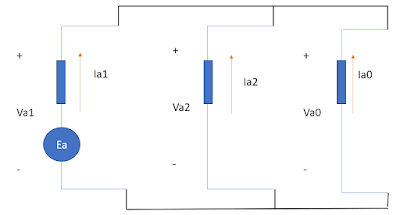Equivalent Sequence Network of DLG Fault

By circuit analysis we can get the equivalent impedance as,

• Zeq = Z1//Z2 + Z0 = j0.1//j0.1 + j0.25 = j0.3 pu
Let the value of Ea = 1 (angle 0).

Therefore, the value of the +s current is,
• Ia1 = Ea / j0.3 pu = -j 3.33 pu

By current division, we can say that the respective values of -s and 0s current as,
• Ia2 = Ia0 = - (-j 3.33/2) = j 1.67 pu
Summary of sequence currents,
• Ia1 = -j 3.33 pu
• Ia2 = j 1.67 pu
• Ia0 = j 1.67 pu
• Fault Current at Phase A, IA = 0.
• Fault Current at Phase B, IB = 5 (angle -30) pu
• Fault Current at Phase C, IC = 5 (angle -150) pu
Apply base values,
• Choose: Sb = 20 MVA and kVb = 13.8 kV, then...
• Ea = 20 MVA/ 20 MVA = 1 (angle 0) per unit.
• Ibase = 20 MVA / (1.73 x 13.8 kV)
• Ibase = 0.837 kA

Therefore the actual Fault Current Values are,
• IA = 0
• IB = 5 x 0.837 kA = 4.185 kA (angle -30)
• IC = 5 x 0.837 kA = 4.185 kA (angle -150)
Voltage Values,

Based on the equivalent sequence network,

• Va2 = Z2 x Ia2 = j 0.1 x (-j 1.67) = 0.167 (angle 0) pu
• Therefore it follows that,  Va2 = Va0 = 0.167 (angle 0) pu
• VA = 0.501 (angle 0) pu
• VB = VC = 0
Apply voltage base,
• VA = 0.501 x (13.8 kV/1.73) = 3.996 kV
• VB = VC = 0

## Unbalanced Fault Analysis: Double Line to Ground Fault

Double line to ground fault when both phases on the three phase line are accidentally connected to the ground. In this case, fault current will flow from the line to the ground within the involved phases, say, Phase B and Phase C.Double Line to Ground Fault - Phase B and Phase C

From this scenario, the system parameters can be considered as follows:

• Fault current at phase A = 0 (since no fault current is flowing in phase A)
• Fault current at phase B = If-B
• Fault current at phase C = If-C.
• Here we can also see that voltages at phase B and phase C will be equal to zero (neglecting ground impedance).

Using the symmetrical components equation matrix formula plus the values we get from the above conditions, we can plot the current equation as follows:Symmetrical Components Matrix Equation of Current for DLG Fault

From this matrix equation, we can get the following values:

• Ia0 + Ia1 + Ia2 = 0 (the sum of all sequence currents is equal to zero)
• IA = 0 (since there is no fault current flowing in Phase A during the fault.

In the same case, the symmetrical components for Voltages is:Symmetrical Components Matrix Equation of Voltage for DLG Fault
From the above matrix equation, we can get the following voltage values:

• Va0 = Va1 = Va2 = Va/ 3
From the obtained voltage and current values, we can demonstrate it using the following sequence network,Equivalent Sequence Network of DLG Fault

This figure satisfies the values obtained based on the given conditions.

For sample calculation, see: Example: Double Line to Ground Calculation

## How to Control Lighting Circuit in 2 or More Locations?

In building wiring, it is important to have an effective and flexible control of the electric lighting system. Irrespective to the type and purpose of the premises, the lighting controls in the building marks the beginning of the efficient use of energy.

For example in staircase, the efficient use of energy starts with the conventional control that enables a person to control the light whether he is in the upper floor or in the lower floor. A person can switch ON the light as soon as he climb the stairs and then he can also switch it OFF when he is already in the upper floor.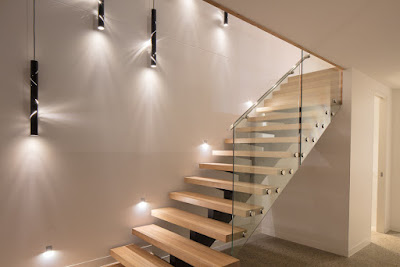Staircase

The same thing happened in the long hallway, there must be a means where the occupant can switch ON and OFF the lights at any strategic point along the hallway.Long Hallway

And so how can we make it possible?

This article covers only the conventional method, without the use of building automation system.

Controlling the light in two locations

Type of switch needed:

• 2 units of 2-way switch (called 3-way switch in North America).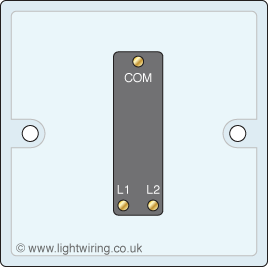Terminals of 2-way switch

As we can see the 2 way switch has 3 terminals namely L1, L2 and common. The connection of the circuit can be done using the following steps:
1. From the source, connect the LINE/HOT conductor to the common terminal of the first switch.
2. From the LINE terminal of the light, use conductor to connect it to the COMMON terminal of second 2- way switch.
3. Connect L1 of the first switch to L1 of the second switch.
4. Connect L2 of the first switch to L2 of the second switch.

This can be best demonstrated in the diagram below,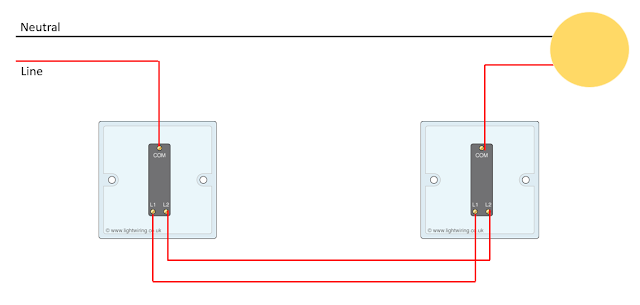Light controlled in 2 locations

Controlling the lighting circuit in 3 or more locations

Type of switch needed:
• 2 units of 2-way switch (called 3-way switch in North America).
• 1 unit - Intermediate Switch (called 4-way switch in North America).
This method can be used efficiently in long hallways where a switching point is a must in a strategic point along the hallway. Here, the use of intermediate switch (4-way switch in North America) is needed.Intermediate Switch

This type of switch has 4 terminals and to be installed in between 2-way switches. For example, if there is a need to control in 3 locations, the 2-way switches will be installed in each end while the intermediate switch will be installed in the middle.

If 4 locations is required then there will be 2 intermediate switch in the middle.

The connection of the circuit can be done using the following steps:
1. From the source, connect the LINE/HOT conductor to the common of the first 2-way switch.
2. From the LINE/HOT terminal of the light, connect it to the common terminal of the second 2-way switch which is to be installed in the other end of the circuit.
3. Connect the intermediate switch in the middle.
This can be best demonstrated in the diagram below,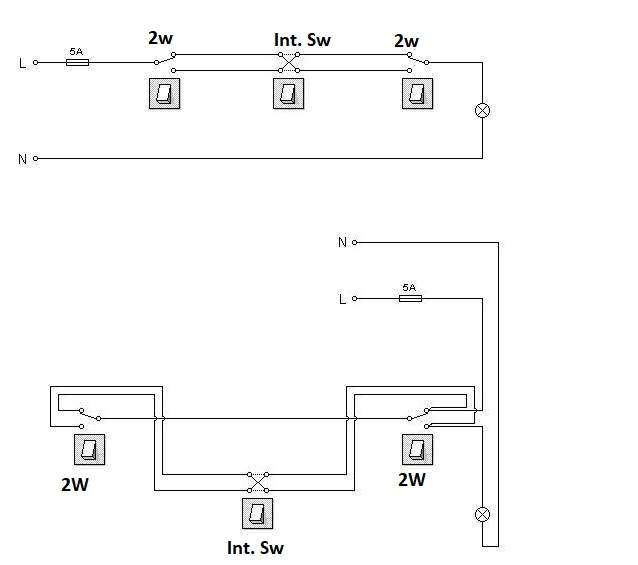Light Controlled in 3 locations.

Note that the 1- unit intermediate switch is just a minimum.  In case the requirement is controlling light in 4 locations, then 2 units intermediate switch are needed.

In general, we need to understand that the Intermediate Switch is always installed in the middle no matter how many units are required. The 2-way switch on the other hand need to be installed in both ends of the circuit.

This can be further explained in the diagram below,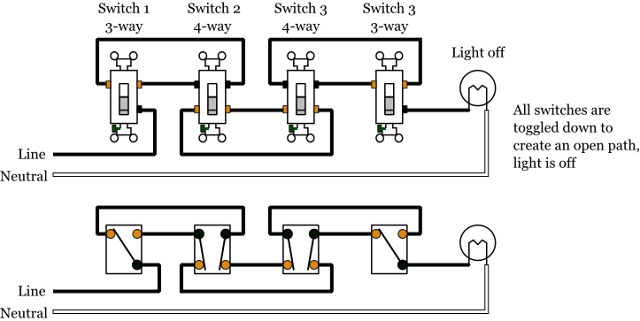Light Controlled in 4 locations.

building wiring, 2 way switch, 3 way switch, intermediate switch, switching control, domestic home wiring, how to control light in 2 locations, how to control lights in 3 locations, how to control light in 4 or more locations.

## Example: Line to Line Fault Calculation

The Line-to-Line fault on a transmission line happens when two current carrying conductors in a three phase system accidentally comes in contact with each other. For this reason, the protective devices of a power system needs to be accurately responsive to avoid severe damage in the system.

See Unbalanced Fault Analysis: Line to Line Fault

For this reason, it is very important to know the procedure of line to line fault calculation in an unbalanced system.

Related articles:

Example:

From the figure given below, assume that the generator is solidly grounded and neglect the fault impedance. Determine the phase currents and phase voltage when Line to Line fault occurs in the system.

Solution:

From the article Unbalanced Fault Analysis: Line to Line Fault, we know that the positive and negative sequence network is connected in parallel and the zero sequence network is not involved in this type of fault.

From the article Unbalanced Fault Analysis: Line to Line Fault, we know that the equivalent positive sequence network is,Positive Sequence Network
While the negative sequence network is,Negative Sequence Network
Connecting this networks in parallel and getting the Thevenin's equivalent looking at the faulted bus and the reference bus, we can get an equivalent impedance of j 0.25 // j 0.1.

Thus, the equivalent sequence network can be simplified as one source of 1 (angle 0) per unit and an equivalent impedance of j 0.71 per unit.

Thus, the positive sequence current will be,

If-1 = 1 (angle 0)/ j 0.71 = -j 1.41 or 1.41 (angle -90) per unit

Since If-1 = (-If-2), we can directly conclude that the negative sequence current is,

If-2 = 1.41 (angle 90) per unit --> See Unbalanced Fault Analysis: Line to Line Fault

Therefore we can summarize the sequence components as follows,
• If-1 = 1.41 (angle -90) per unit (positive sequence current)
• If-2 = 1.41 (angle 90) per unit (negative sequence current)
• If-0 = 0 (zero sequence network is not involved in Line to Line fault)
By using sequence to phase matrix formula, we can get the values of fault current as,
• Fault current at phase A = 0.
• Fault current at phase B = 2.442 (angle 180) pu
• Fault current at phase C = 2.442 (angle 0) pu
Consider base values,

Choose: Sb = 20 MVA and kVb = 13.8 kV

then,
Ea = 20 MVA/ 20 MVA = 1 (angle 0) per unit.
Ibase = 20 MVA / (1.73 x 13.8 kV)
Ibase = 0.837 kA

Therefore the actual values of fault currents are,
• Fault current at phase A = 0.
• Fault current at phase B = 2.04 kA (angle 180)
• Fault current at phase C = 2.04 kA (angle 0)
Voltage values,
Analyzing positive sequence network equivalent,
Vf-1 = 1 (angle 0) - (If-1) * (Z1) = 1 - (-j 1.41) (j 0.25) = 0.6475 (angle 0) (+ sequence voltage)

since Vf-1 = Vf-2; therefore Vf-2 = 0.6475 (angle 0) (neg. sequence voltage) See Unbalanced Fault Analysis: Line to Line Fault

Applying the formula of sequence to phase values matrix we can get:
• Voltage at phase A = 1.295 (angle 0) pu
• Voltage at phase B = 0.647 (angle 180) pu
• Voltage at phase C = 0.647 (angle 180) pu
Applying base values,

Vbase = 13.8 kV/ 1.73

Therefore the actual voltage values are,
• Voltage at phase A = 10.31 kV (angle 0)
• Voltage at phase B = 5.15 kV (angle 180)
• Voltage at phase C = 5.15 kV(angle 180)

For more details See Unbalanced Fault Analysis: Line to Line Fault

power system analysis, fault calculation, unbalance fault, short circuit analysis

## Unbalanced Fault Analysis: Line to Line Fault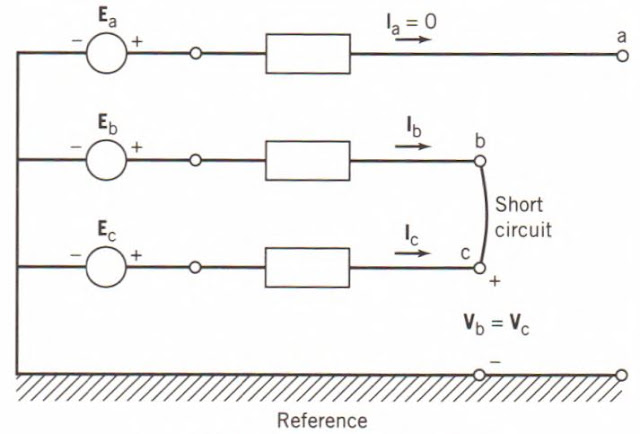Line to Line Fault

Line to line fault happens when two phases of the three phase line accidentally connected each other. In this case, the fault current will flow for both phases involved. From the given diagram, the fault occurs in phases B and C while phase A remain unfaulted.

From this scenario, the system parameters can be considered as follows:

• Fault current at phase A = 0 (since no fault current is flowing in phase A)
• Fault current at phase B = If-A
• Fault current at phase C = If-C.
• Here we can also see that voltages at phase B and phase C will be equal during fault, thus VB = VC.

Using sequence network matrix formula, we can plot the given values as follows.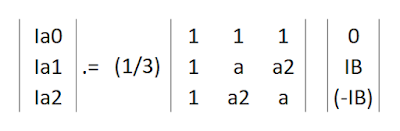Symmetrical Components Matrix Formula in Line to Line Fault
From this matrix equation, we can get a value of,
• Ia0 = 0
• Ia1 = - Ia2
Which means, the zero sequence current in a Line to Line fault is not involved while the positive sequence current and negative sequence current is opposing each other.

In the sames sense, the matrix formula can also gives us the value of the voltages,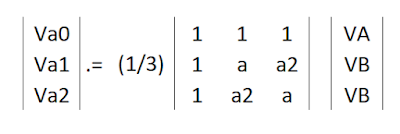Symmetrical Components Matrix Formula of Line to Line Fault

Note that in this case, VB = VC. By manipulating equations we can get Va1 = Va2.

From the result of two matrix equations involving current and voltage, we can develop a sequence network which describes as that the positive and negative sequence is in parallel with each other.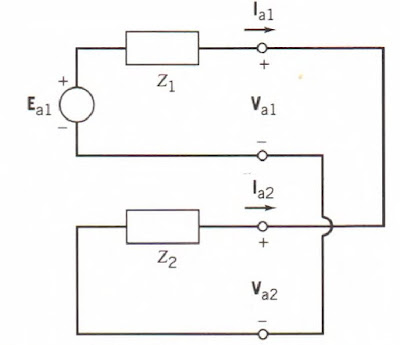Equivalent Sequence Network for Line to Line Fault

This figure satisfies the equation Va1 = Va2 and Ia1 = (-Ia2).

For sample calculation, see the following article Example: Line to Line Fault Calculation

## Example: Single Line-to-Ground Fault Calculation

The single line-to-ground fault on a transmission line happens when one conductor accidentally to the ground or comes in some cases in contact with the neutral conductor. For this reason, the protective devices of a power system needs to be accurately responsive to avoid severe damage in the system.

See Unbalanced Fault Analysis: Single Line to Ground Fault

Indeed, it is very important to know the procedure of single line to ground fault calculation.

Related articles:

• Double Line to Ground Fault
• Line to line fault
• Symmetrical three phase fault

Example:
Assumed that the generator below is solidly grounded and neglect the fault impedance. Determine the phase currents and phase voltage at faulted location.Generator

Solution:

Compute the base value:
Choose: Sb = 20 MVA and kVb = 13.8 kV
then,
Ea = 20 MVA/ 20 MVA = 1 (angle 0) per unit.
Ibase = 20 MVA / (1.73 x 13.8 kV)
Ibase = 0.837 kA

Develop positive sequence network:System Positive- Sequence Network Equivalent
where,
• Ea = the generator EMF, which has a value of 1 (angle 0) per unit.
• Xd" = the positive sequence impedance of transformer (in subtransient state)
• If-1 = positive sequence current.
Develop negative sequence network: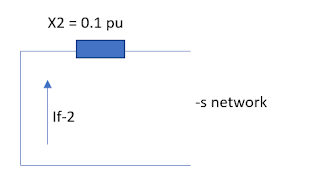System Negative- Sequence Network Equivalent
where,
• If-2 = negative sequence current
• X2 = negative sequence reactance

Develop zero sequence network:System Zero-Sequence Network Equivalent
where,
• If-0 = zero sequence current
• X2 = zero sequence reactance
By principle, the single line to ground fault will develop and equivalent network where all sequence networks are connected in series.

then, If-1 = 1 (angle 0)/ (Z0 + Z1 + Z2) = -j 2.22 pu or 2.22 (angle -90)
also it means that, If-2 = If-0 = - j 2.22 pu

Multiplying the base value

Ibase = 0.837 kA
then, If-1 = 2.22 (angle -90) * (0.837 kA) = 1.86 kA (angle -90) --> actual value
Since all sequence currents are equal, thus:
• If-1 = 1.86 kA (angle -90) --> + S
• If-2 = 1.86 kA (angle -90)  --> -S
• If-0 = 1.86 kA (angle -90) --> 0S
Applying the formula of sequence to phase values matrix we can get:
• Fault current at A = 5.58 kA (angle -90 degrees)
• Fault current at B = 0
• Fault current at C = 0
The zero values of phases B and C confirmed that no fault current flows from it during fault condition.

Computing Voltage at the faulted point,

Looking at this diagram, the voltage values of the un-faulted phases (phase B and C) are the only one that has a value while phase A (the faulted phase), its voltage is equal to zero (neglecting impedance).

Thus,

• Voltage at phase A = 0
• Voltage at phase B = Vb
• Voltage at phase C = Vc.
Analyzing positive sequence network equivalent,
• Vf-1 = 1 (angle 0) - (If-1) * (Z1) = 1 - (-j 2.22) (j 0.25) = 0.445 (angle 0) (+ sequence voltage)
Analyzing negative sequence network equivalent,
• Vf-2 = 0 - (If2) * (Z2) = 0- (-j2.22) * (j0.1) = 0.222 (angle 180) ( neg. sequence voltage).
Analyzing zero sequence network equivalent,
• Vf-0 = 0 - (If-0)* (Z0) = 0- (-j 2.22) * (j0.1) = 0.222 (angle 180) (zero sequence voltage).
Applying the formula of sequence to phase values matrix we can get:
• Voltage at phase A = 0
• Voltage at phase B = 0.667 (angle -120) pu
• Voltage at phase C = 0.667 (angle 120) pu
Applying base value,

Vbase = 13.8 kV/ 1.73

Therefore, the actual values of phase voltages are,
• Voltage at phase A = 0
• Voltage at phase B = 5.31 kV (angle -120)
• Voltage at phase C = 5.31 kV (angle 120)

## Unbalanced Fault Analysis: Single Line to ground fault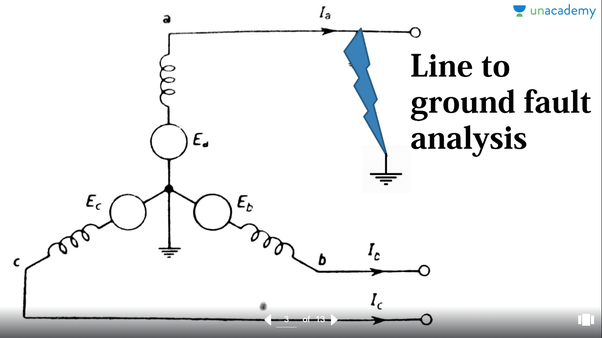Photo Source: UNACADEMY

In three phase system, Single Line to Ground Fault happens when one of the lines or phase of the system is accidentally connected to ground. In this case unbalanced voltage will be developed in the system as well as unbalanced current.

See Sample of Single Line to Ground Fault Calculation

By principle, all sequence components i.e. positive, negative and zero sequence components will be connected in series. See article: How to Develop Sequence Network in an Unbalanced System?

In this regard, the above diagram can be further represented below.Single Line to Ground Fault

From the figure above, we can see that the fault current is equal to Ia. On the other hand, Ib and Ic will be both zero since there will be no fault current that will flow from that phases.

Thus the fault currents in this case can be summarize as:
• Fault Current at A = Ia
• Fault Current at B = 0
• Fault Current at C = 0
Using the principle of symmetrical components we can get the corresponding sequence values. (See article: What is Symmetrical Components?)

• I0 = IA
• I1 = IA
• I2 = IA
Therefore we can present the sequence network as follows:

In this case, all sequence component networks i.e. the positive, negative and zero sequence are connected in series. This will affirm the equality of all sequence currents.

See Sample of Single Line to Ground Fault Calculation

## How to Develop Sequence Network in an Unbalanced Faulted System?

Fault analysis is very important part of power system study. Without fault analysis, we cannot determine the exact specifications of different protective devices in the system. Accordingly, fault analysis can be more tedious when dealing with unbalanced system. The calculation of fault currents in an unbalanced system starts from the development of sequence network.

For this reason, the method of symmetrical components are widely used to carry out the calculations. Although there are already computer software that can perform in behalf of the manual calculation, still, it is necessary to understand the fundamental concept of fault analysis.

From the previous article of symmetrical components, it is explained how an unbalanced vectors are converted into three sets of equal vectors namely: the positive sequence, negative sequence and zero sequence components.

Accordingly, these values have also equivalent sequence networks such as the sequence network of transformers, and others.

There are three types of unbalanced fault in three phase system:
• Single line to ground fault - this fault happens when one phase of the system is accidentally connected to the ground.
• Line-to- line fault - this fault happens when two live conductors accidentally connected with each other.
• Double- line- to ground fault - this happens when two phases are accidentally connected to the ground.

Once the sequence networks for the system are defined, the way they are connected is dependent on the type of fault. Sequence network connections for common shunt fault types are shown.

1. Single line to ground fault: In this type of fault, the positive, negative and zero sequence components are connected in series. It follows that, all sequence currents have similar value i.e. I1 = I2 = I0.

2. Line-to- line fault or Phase to Phase Fault:  In this type of fault, the zero sequence network is non-existent. The only networks involved are the positive and negative sequence respectively and the two networks are connected in parallel. As we can see in the figure below, the negative sequence current is opposite t the positive sequence current. I1 = - I2.

3. Double Line- to- round fault: In this type of fault, all sequence networks are connected in parallel. By simple Kircchoff's Current Law in the top node we can find that I1 + I2 + I0 = 0.

The rest of the analysis can be done using circuit analysis with complex quantities.

See more,

## How to Develop Zero- Sequence Network of Transformer?

Like any other elements of power system, transformer can also be represented by its corresponding sequence network. However, the characteristic of transformer is unique which is dependent on how it is banked. The transformer representation in its zero sequence network can be more complex compare with its positive and negative components.

Below are the sequence network of a three phase transformer based on it's banking connection.

1. Y-Y Transformer with grounding

When a transformer has at least two grounded-wye windings, zero-sequence current can be transformed between the grounded-wye windings. The I0 currents will add up to 3I0 in the neutral and return through ground or the neutral conductor. The I0 currents will be transformed into the secondary windings and flow in the secondary circuit. Any impedance between the transformer neutral points and ground must be represented in the zero-sequence network as three times its value to correctly account for the zero-sequence voltage drop across it. Below on the left is a three-phase diagram of a grounded-wye, grounded-wye transformer connection with its zero-sequence network model on the right. Notice the resistance in the neutral of the secondary winding is modeled by 3R in the zero-sequence network model.Zero-Sequence model of Y-Y transformer (both grounded)

2. Delta-Wye (wye is grounded)

When a transformer has a grounded-wye winding and a delta winding, zerosequence currents will be able to flow through the grounded-wye winding of the transformer. The zero-sequence currents will be transformed into the delta winding where they will circulate in the delta without leaving the terminals of the transformer. Because the zero-sequence current in each phase of the delta winding is equal and in phase, current does not need to enter or exit the delta winding. Below on the left is a three-phase diagram of a grounded-wye-delta transformer connection with its zero-sequence network model on the right.Zero Sequence model of Delta-Wye (grounded) transformer

As we can see, all WYE connections are grounded. In case, it is solidly grounded and no impedance exist in the ground conductor then simply eliminate it and replace it with short circuit (zero impedance).

All other models can be represented by the figure below,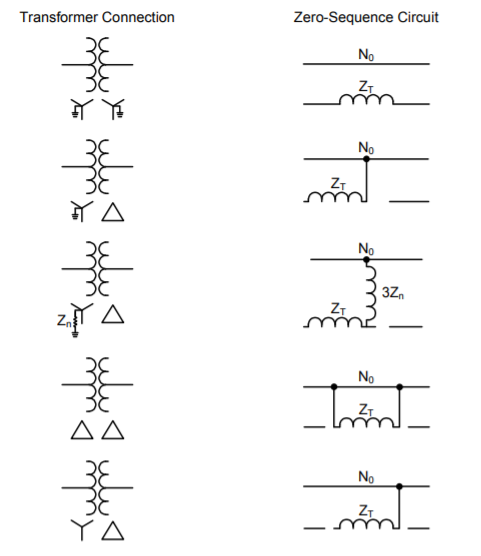General Representation of Transformer Zero-Sequence Network

As shown from the diagram above, there is no zero sequence current that will flow to the reference bus of faulted system for delta connection and Wye (ungrounded).

This diagram can be explained by the rules applied on the zero-sequence networks of transformer.
1. When magnetizing current is neglected, transformer primary would carry current only if there is current flow on the secondary side.
2. Zero sequence currents can flow in the legs of wye connection only if it is grounded and provide effective path to the ground.
3. No zero sequence currents can flow in the lines of delta connection as no return path is avaiable for these currents.

## What is symmetrical components?

The method of symmetrical components is used to analyze unbalance system by converting it to three sets of balanced phasors.

Supposed there is an unbalanced three phase current of Ia, Ib and Ic. These values can be represented by its corresponding symmetrical components such as the following:

• positive sequence: I1
• negative sequence: I2
• zero sequence: I0
To find the values of the above components, the following matrix formula will be used.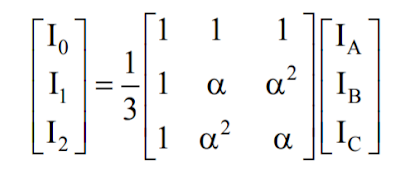Phase Values to Sequence Components

where:

α = 1 ë 120 degrees.

From the above formula we can get,

I0 = 1/3 (IA + IB + IC)

I1 = 1/3 (IA + IB*a + IC*a*a)

I2 = 1/3 (IA + IB*a*a + IC*a)

In this diagram, the unbalanced phasors are broken down into balanced phasors. As seen from diagram above, Va1 (positive sequence) rotates counter-clockwise with a sequence of Va1, Vb1 and Vc1, these vectors are of equal magnitude and 120 degrees apart.

In the same situation, Va2 (negative sequence) also rotates counter- clockwise with a sequence f Va2, Vc2 and Vb2. Negative sequence vectors have also equal magnitude and 120 degrees apart.

Here, the difference between the positive sequence and negative sequence can be distinguished by looking at the sequence and order of vector rotation.

Lastly, Va0 (zero sequence) have vectors of equal magnitude but these vectors are in-phase with each other. Meaning there is no angle of displacement between vectors.

Similarly, given symmetrical components values, it can be converted back to phase values using the following matrix formula: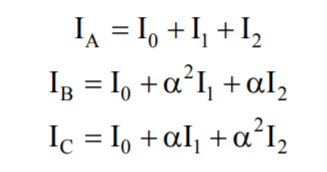Sequence components to Phase Values Matrix

Example:
The electrical system has three unbalanced current of Ia = 100 ë50 A; Ib = 75 ë35 A; Ic= 65 ë45. Find the corresponding symmetrical component values?
From the formula given above,
I0 =  1/3 (Ia + Ib + Ic)
I1 = 1/3 (Ia + Ib * α + Ic * α * α)
I2 = 1/3 (Ia + Ib * α * α + Ic α )
note: α = 1 ë 120 degrees.
Calculating values, the results are:
I0 = 80 ë44 A.
I1= 16 ë74 A
I2= 7 ë 77 A.

The symmetrical components are very important in calculating fault analysis. This is because all parts of electrical power system such as motors, generators, transformers and transmission lines can be represented by its corresponding sequence network components.